# Oracle Aggregate Functions - oracle tutorial - sql tutorial

## What is Aggregate Functions in oracle ?

• Aggregate functions return a single value based on groups of rows, rather than single value for each row.
•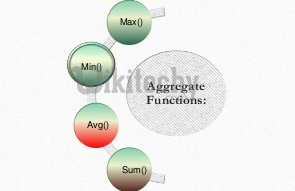• The important Aggregate functions are :
• Avg
• Sum
• Max
• Min
• Count
• Stddev
• Variance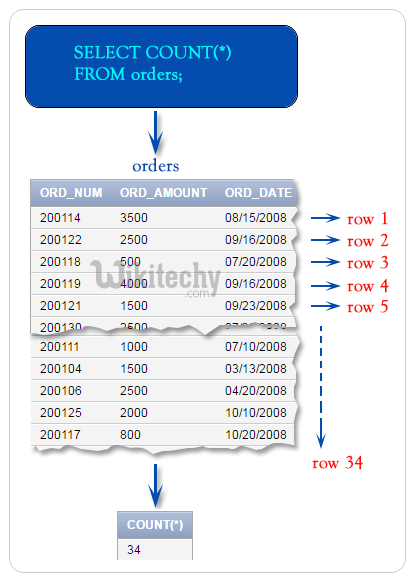Oracle sql count functions• Here we are showing the table “wikitechy_char” with its fields before the AGGREGATE FUNCTION has been executed.

## AVG:

• Returns the average value of expression.

## Example: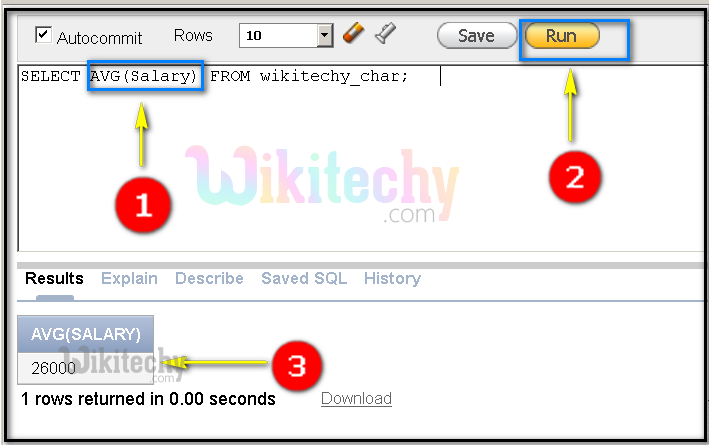• AVG(Salary) function is being used to show the average of salary from the table “wikitechy_char”.
• Click on the RUN button to execute the query.
• Here we can see the output of the AVG(Salary) function as 26000.

## SUM:

• Returns the sum value of expression.

## Example: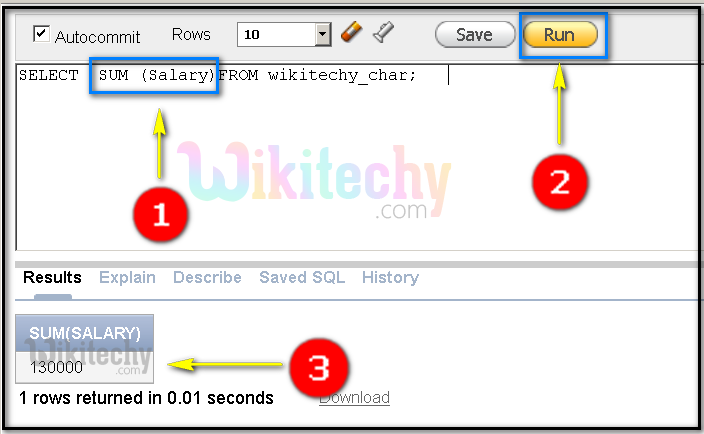• SUM (Salary) function is being used to show the sum of salary from the table “wikitechy_char”.
• Click on the RUN button to execute the query.
• Here we can see the output of the SUM(Salary) function as 130000.

## MAX:

• Returns maximum value of expression.

## Example: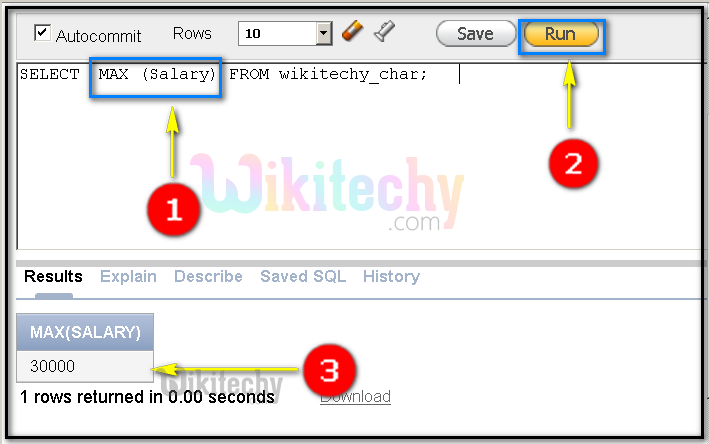• MAX (Salary) function is being used to show the maximum salary from the table “wikitechy_char”.
• Click on the RUN button to execute the query.
• Here we can see the output of the MAX(Salary) function as 30000.

## MIN:

• Returns minimum value of expression.

## Example: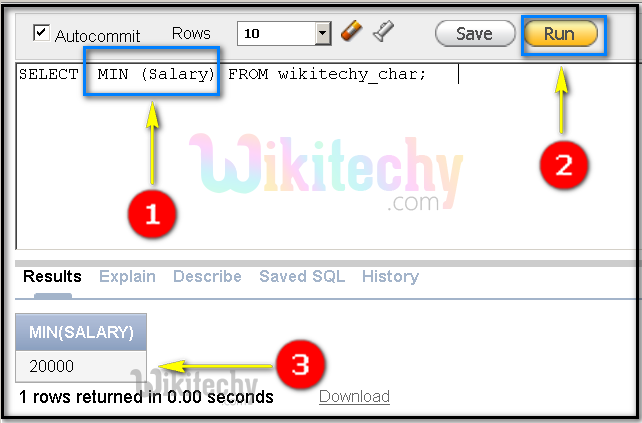• MIN (Salary) function is being used to show the minimum salary from the table “wikitechy_char”.
• Click on the RUN button to execute the query.
• Here we can see the output of the MIN(Salary) function as 20000.

## COUNT:

• Returns the number of rows in the query. If you specify expression then count ignore nulls.
• If we specify the asterisk (*), this function returns all rows, including duplicates and nulls.
• COUNT never returns null.

## Example: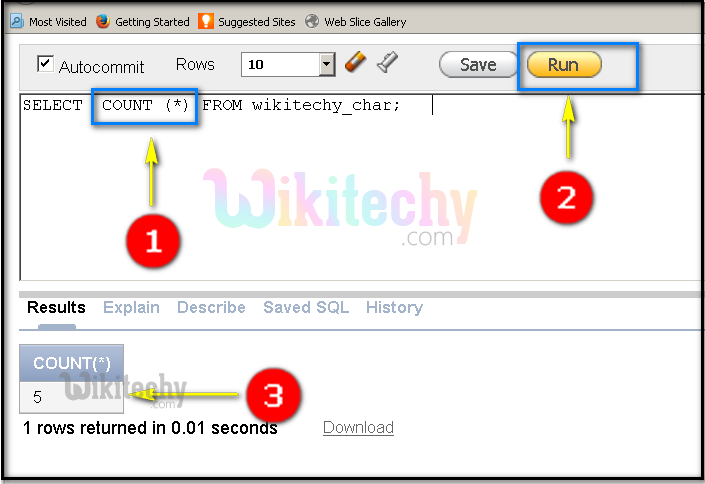• COUNT (*) function is being used to show the number of rows in the table “wikitechy_char”.
• Note: Asterisk (*), this function returns all rows,
• Click on the RUN button to execute the query.
• Here we can see the output of the COUNT(*) function as 5 .
• COUNT (Salary) function is being used to show the number of rows in the table “wikitechy_char”.
• Click on the RUN button to execute the query.
• Here we can see the output of the COUNT(Salary) function as 5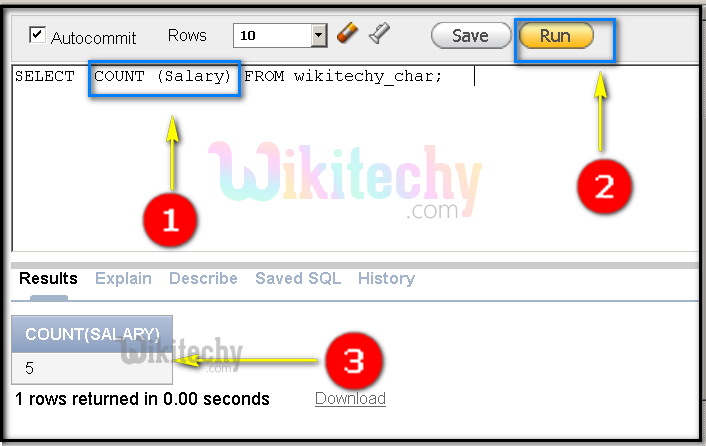• COUNT (Salary) function is being used to show the number of rows in the table “wikitechy_char”.
• Click on the RUN button to execute the query.
• Here we can see the output of the COUNT(Salary) function as 5

## STDDEV:

• STDDEV returns sample standard deviation of expression, a set of numbers.

## Example: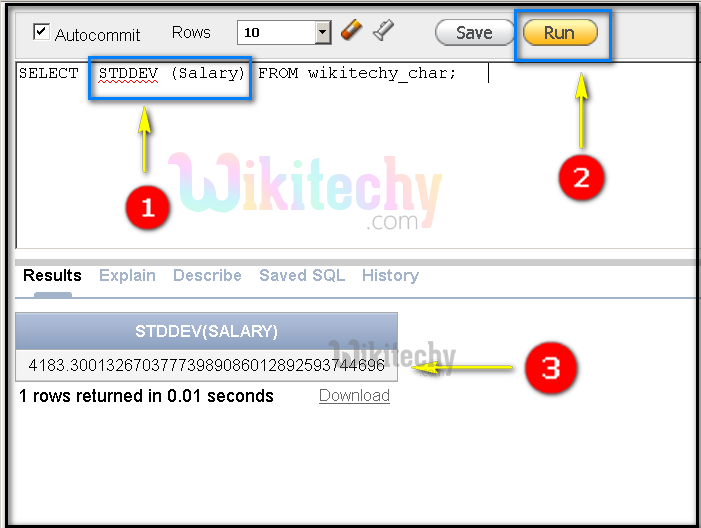• STDDEV (Salary) function is being used to show the standard deviation in the table “wikitechy_char”.
• Click on the RUN button to execute the query.
• Here we can see the output of the STDDEV(Salary) function as 4183.300.

## VARIANCE:

• Variance returns the variance of expression.

## Example: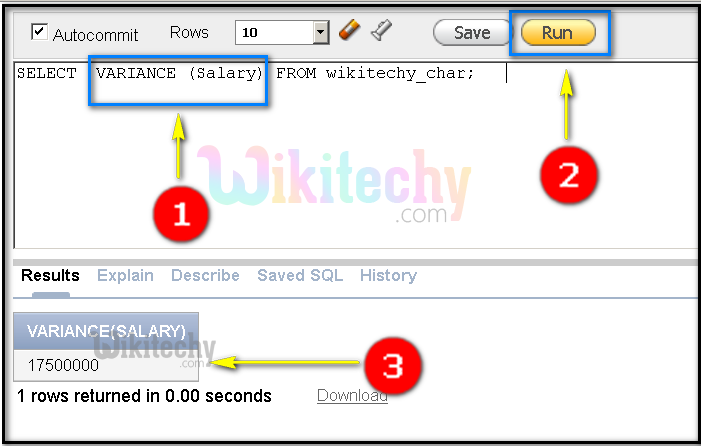• VARIANCE (Salary) function is being used to show the variance of salary in the table “wikitechy_char”.
• Click on the RUN button to execute the query.
• Here we can see the output of the VARIANCE (Salary) function as 1750000.

## Oracle Exercise Aggregate Function Example